# 切空间与切丛

### “向量空间”, “流形”之概念

$$\overrightarrow{v} = \begin{pmatrix} x_1\\ x_2\\ \vdots\\ x_n \end{pmatrix}$$

$$x:=(x_1, x_2, \cdots x_n).$$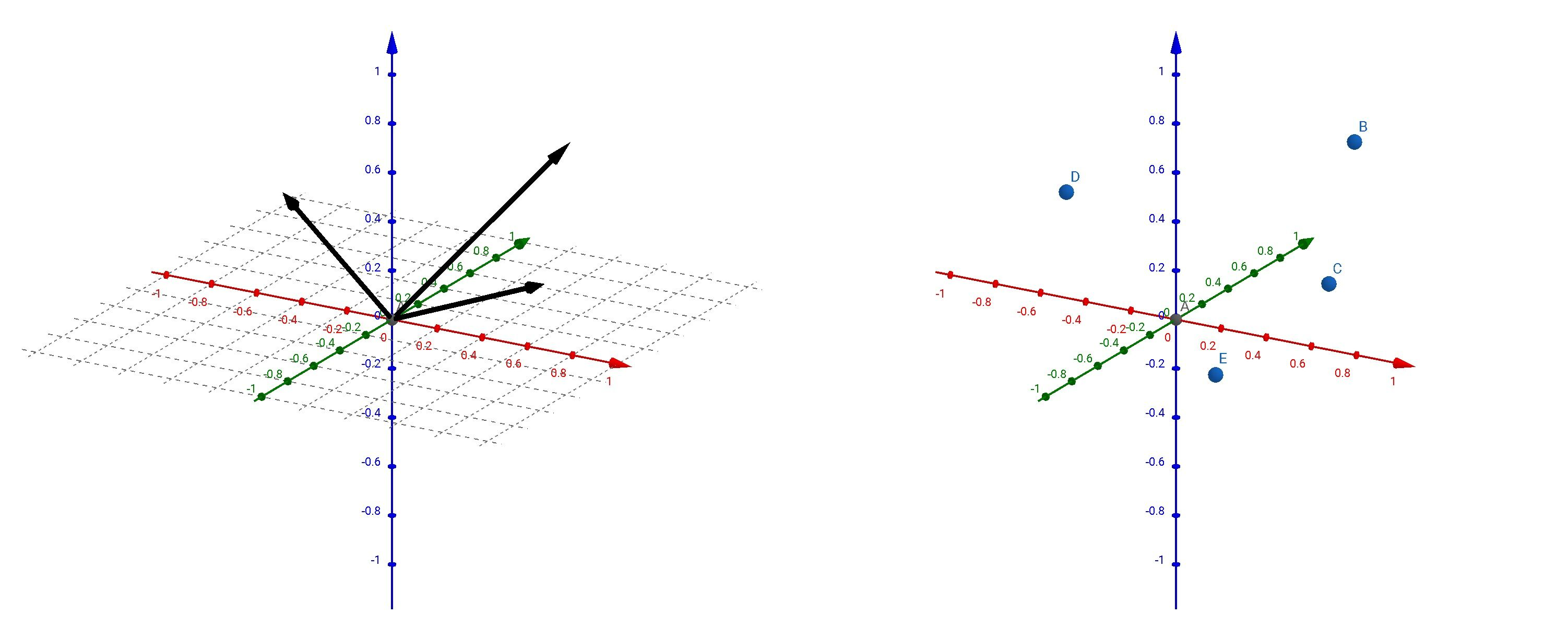### 切空间 (Tangent Space)

• 平面上的给出一条光滑曲线, 对于其上的给定的一个点 $p$ 可以给出相应的曲线的切线. 由点 $p$ 出发终点位于这条切线上的向量, 自然称为曲线在这一点处的切向量.
显然, 曲线在点 $p$ 处的全部切向量构成一个向量空间 $\mathbb{R}$.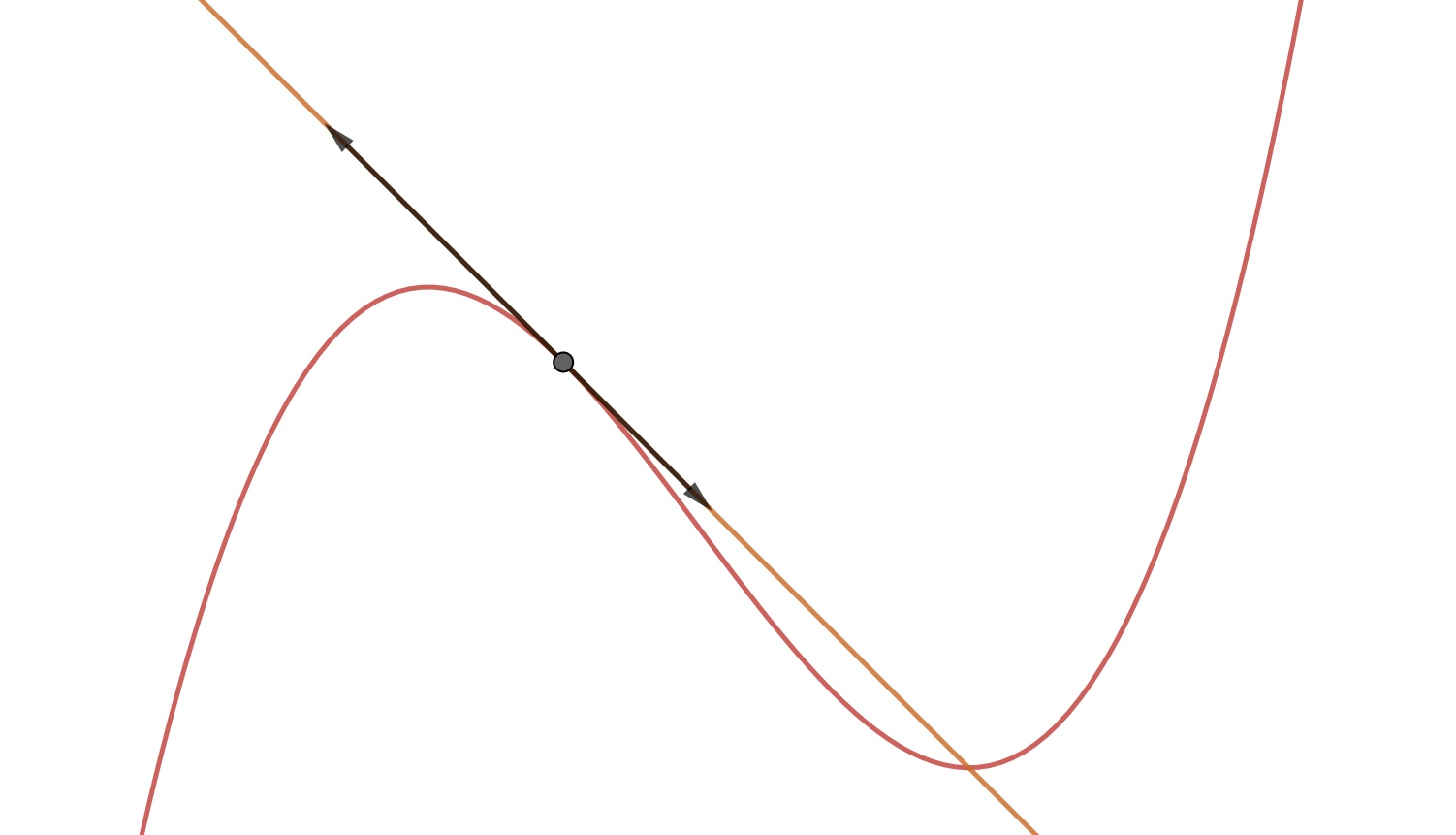• 球面 $S^2$ 是一个 2 维流形, 对于其上的给定的一个点可以给出相应的切平面, 由该点出发终点位于这个切平面上的向量, 也可以称为切向量. 显然, 球面在该点处的全部切向量构成一个向量空间 $\mathbb{R}^2$, 每个切向量也对应着球面上一个大圆, 是相应的大圆在这一点处的切向量.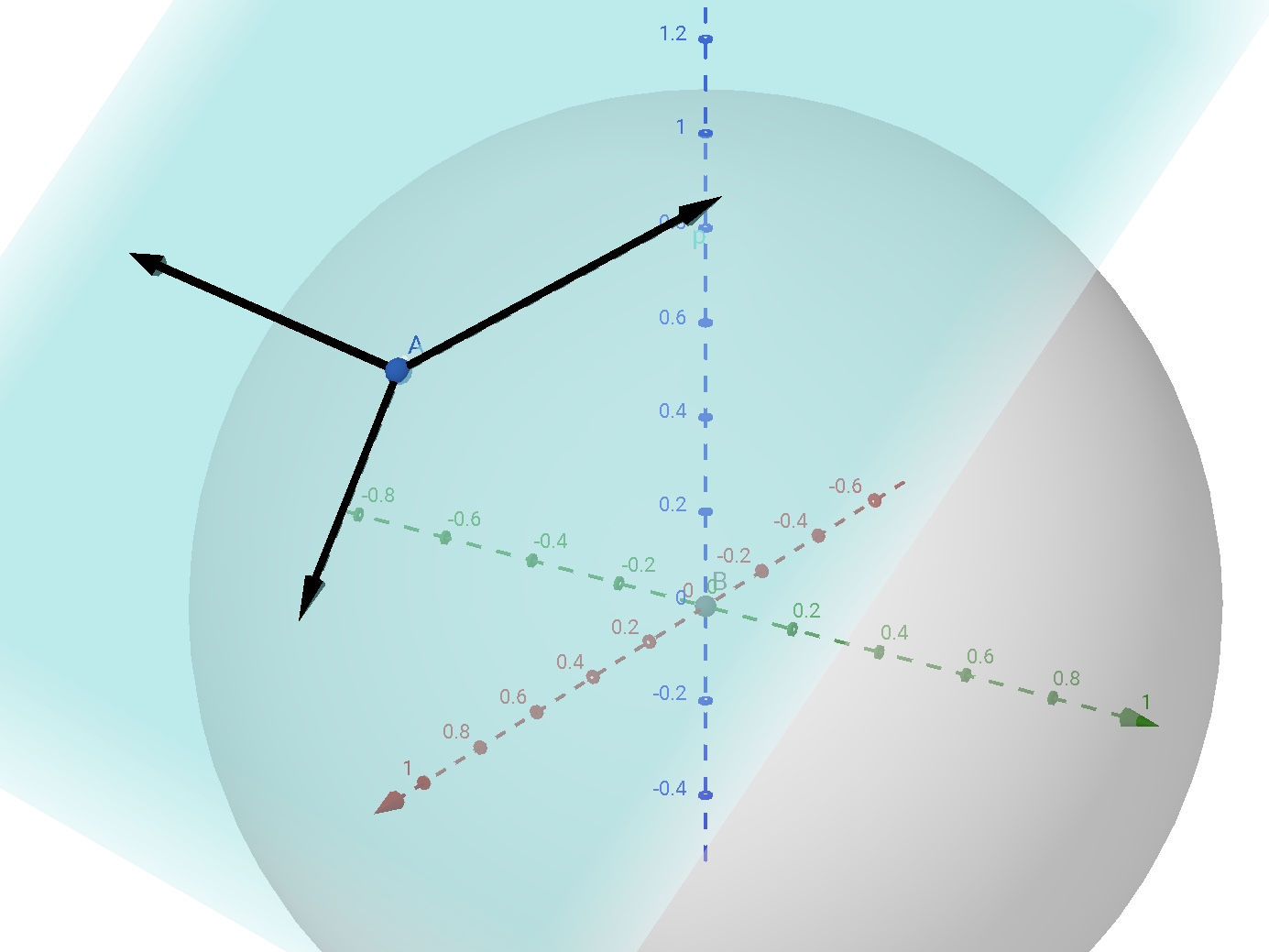【定义】光滑流形 $M$ 在一点 $p$ 处的切空间, 是由 $M$ 上所有通过点 $p$ 的曲线在 $p$ 处的切向量所构成的空间. 用符号 $TM_{p}$ 或 $T_p M$ 表示.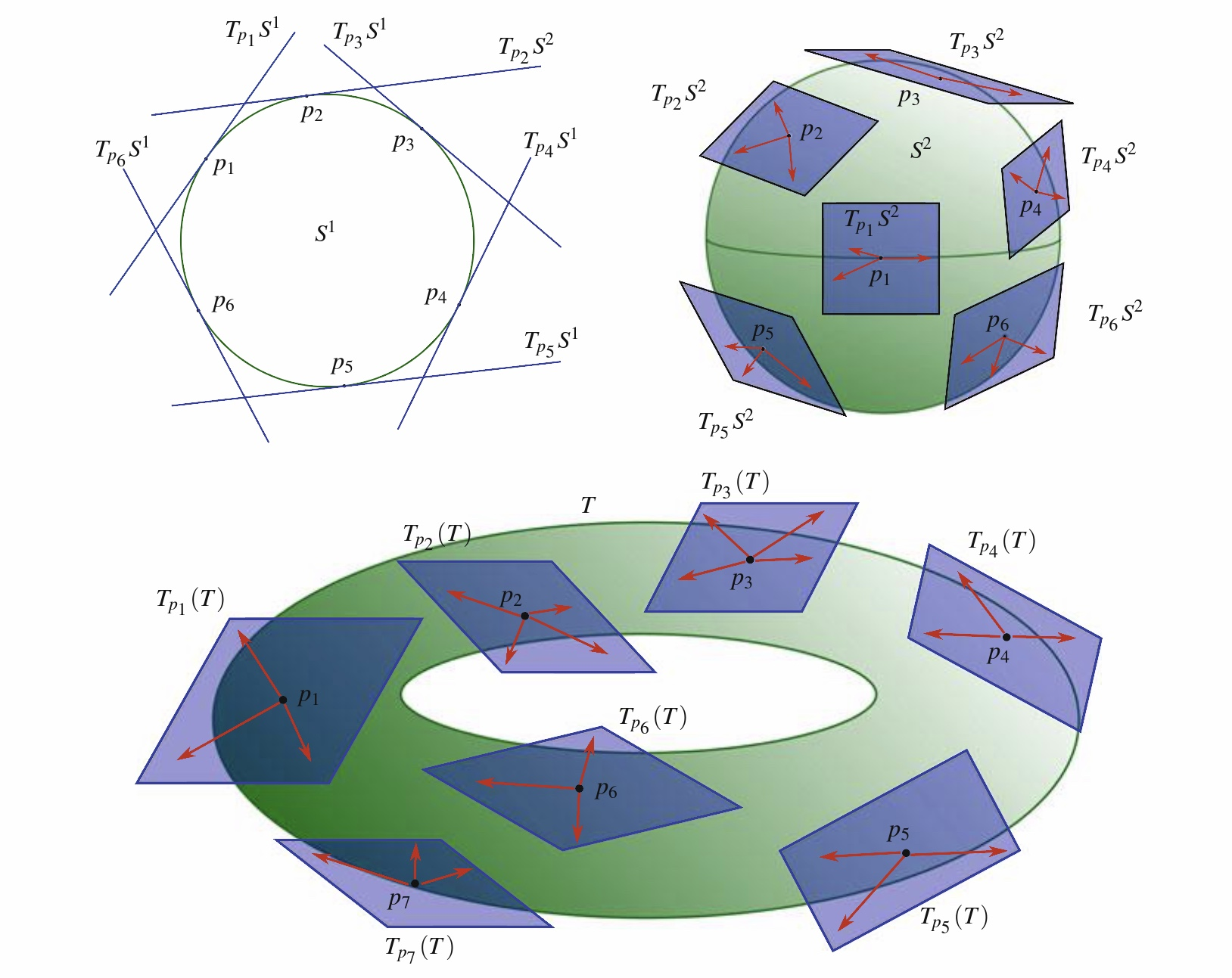### 切丛 (tangent bundle)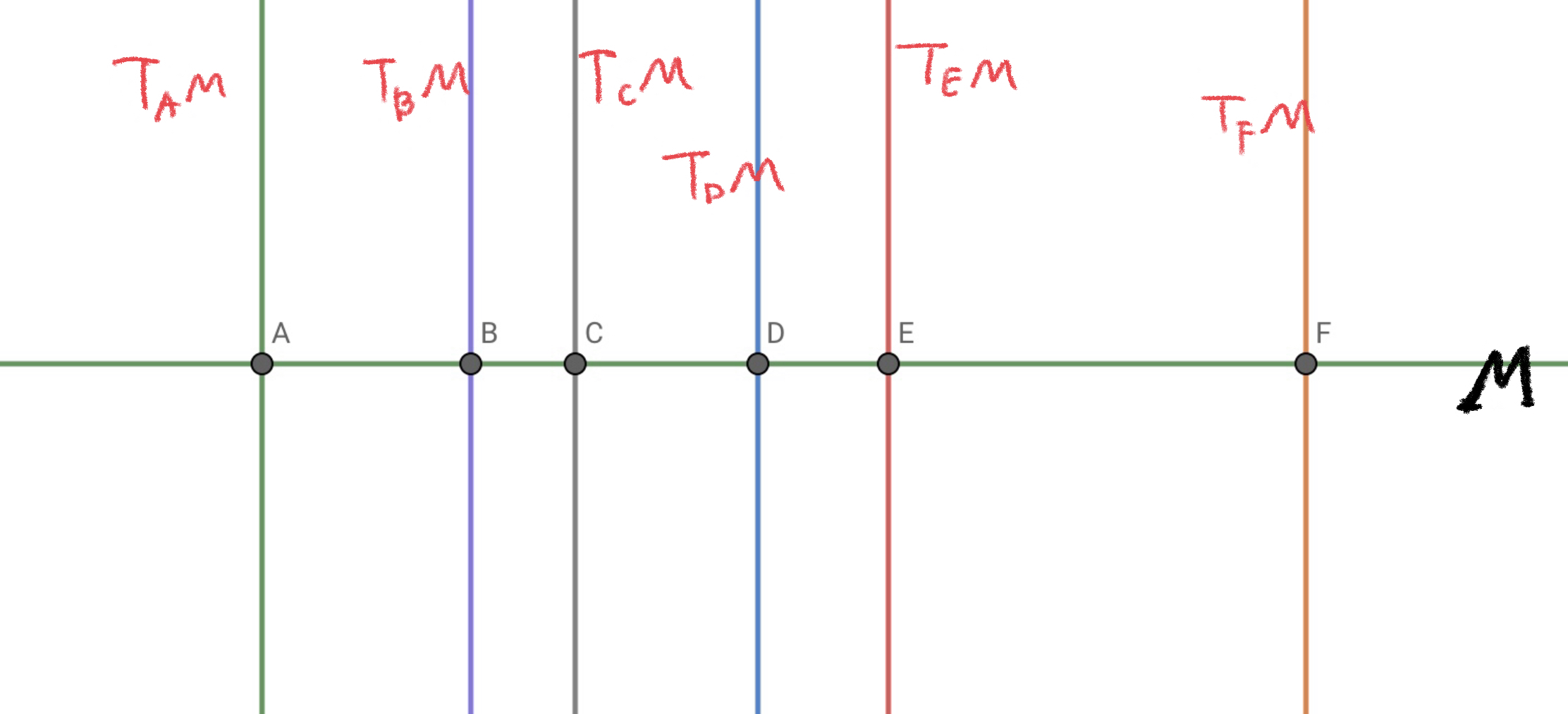$n$ 维流形 $M$ 的切丛 $TM$ 是 $2n$ 维的.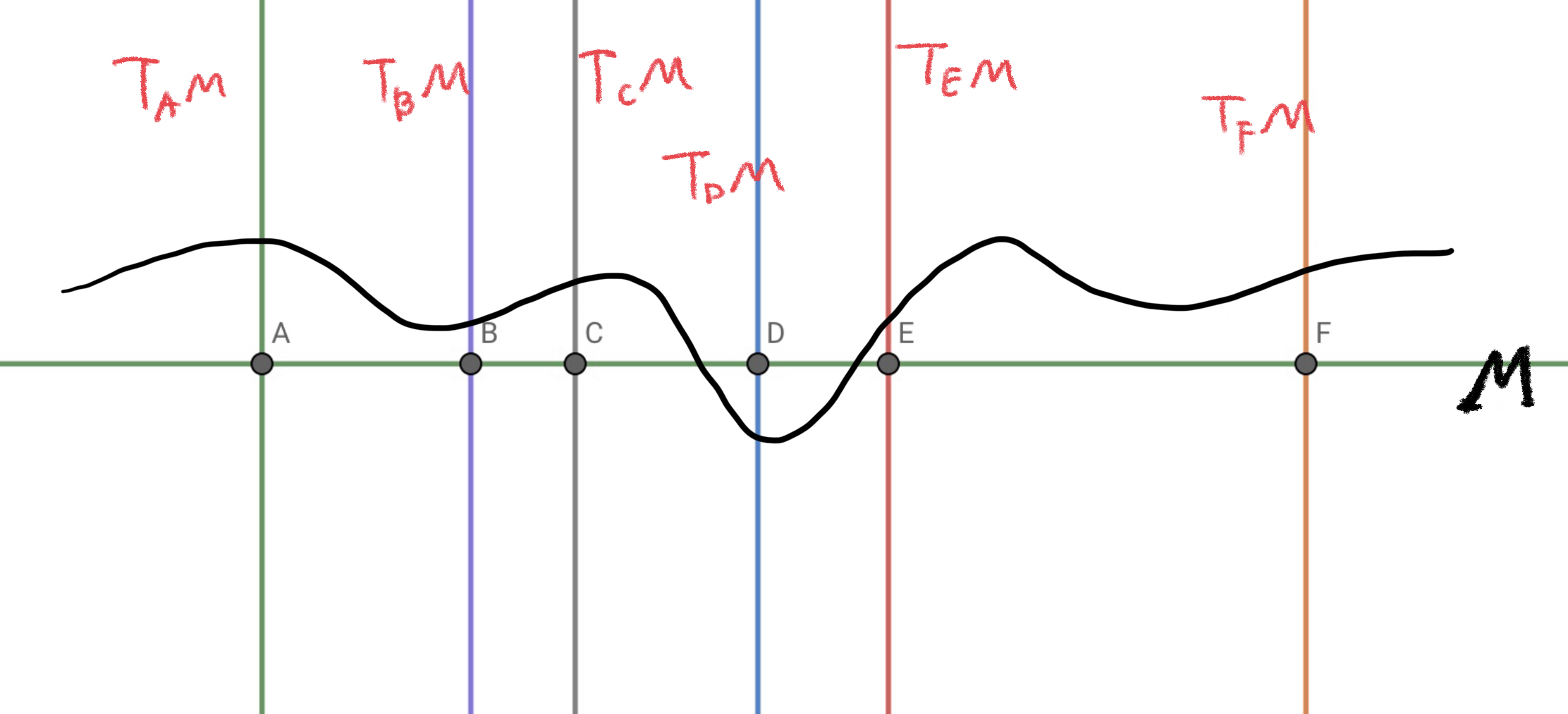Zengfk

2019-09-26

2019-09-29